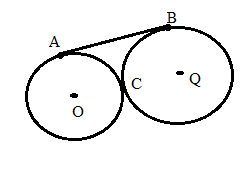Chapter 6.3, Problem 37EElementary Geometry For College St...

7th Edition
Alexander + 2 others
ISBN: 9781337614085

Solutions

Chapter
SectionElementary Geometry For College St...

7th Edition
Alexander + 2 others
ISBN: 9781337614085
Textbook Problem

Given: A B ¯ is an external tangent to ⊙ O and ⊙ Q at points A and B; radii lengths for ⊙ O and ⊙ Q are 4 and 9.Find: AB(HINT: The line of centers O Q ¯ contains point C, the point at which ⊙ O and ⊙ Q are tangent.)

To determine

To find:

The find the value of AB.

Explanation

Given that, AB¯ is an external tangent to O and Q at points A and B; radii lengths for O and Q are 4 and 9.

The line of centers OQ¯ contains the point C in which the circles are internally tangent. Then draw the tangent and join O, Q with P.

The diagrammatic representation is given below,

From the above graph PA¯,PC¯ tangents from the exterior point P to circle O. That is, PO¯ bisects APC therefore, PA=PC.

From the above graph PB¯,PC¯ tangents from the exterior point P to circle Q. That is, PQ¯ bisects CPB therefore, PC=PB.

Then add the angles to get the following,

mOPQ=mOPC+mQPC=mAPC2+mCPB2=mAPC+mCPB2=90+90"

Still sussing out bartleby?

Check out a sample textbook solution.

See a sample solution

The Solution to Your Study Problems

Bartleby provides explanations to thousands of textbook problems written by our experts, many with advanced degrees!

Get Started

Find more solutions based on key concepts